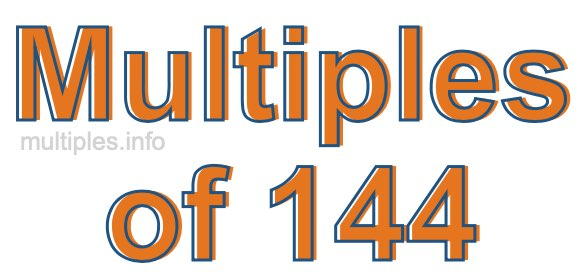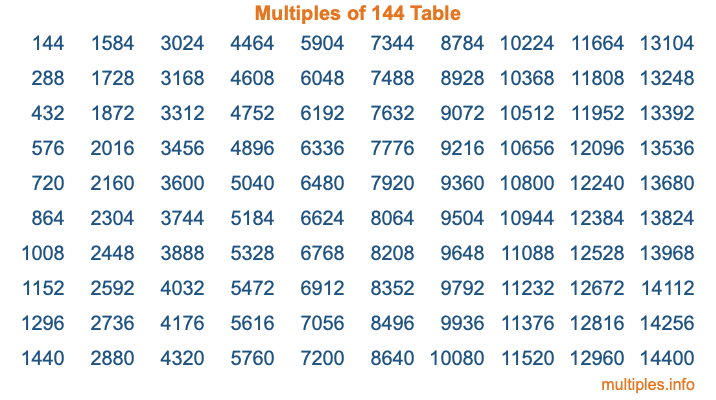Multiples of 144Welcome to the Multiples of 144 page. Here we will first teach you everything you will ever need to know about the multiples of 144, and then give you a study guide summary of everything we taught you to make sure you remember it all. Use this page to look up facts and learn information about the multiples of 144. This page will make you a multiples of one hundred forty-four expert!

Definition of Multiples of 144
Multiples of 144 are all the numbers that when divided by 144 equal an integer. Each of the multiples of 144 are called a multiple. A multiple of 144 is created by multiplying 144 by an integer.

Therefore, to create a list of multiples of 144, you start with 1 multiplied by 144, then 2 multiplied by 144, then 3 multiplied by 144, and so on for as long as you want. Thus, the list of the first five multiples of 144 is 144, 288, 432, 576, and 720. To see a larger list of multiples of 144, see the printable image of Multiples of 144 further down on this page. We also have a category where you can choose any nth multiple of 144.

Multiples of 144 Checker
The Multiples of 144 Checker below checks to see if any number of your choice is a multiple of 144. In other words, it checks to see if there is any number (integer) that when multiplied by 144 will equal your number. To do that, we divide your number by 144. If the the quotient is an integer, then your number is a multiple of 144.

Is  a multiple of 144?

Least Common Multiple of 144 and ...
A Least Common Multiple (LCM) is the lowest multiple that two or more numbers have in common. This is also called the smallest common multiple or lowest common multiple and is useful to know when you are adding our subtracting fractions. Enter one or more numbers below (144 is already entered) to find the LCM.

Check out our LCM Calculator if you need more details about the Least Common Multiple or if you need the LCM for different numbers for adding and subtraction fractions.

nth Multiple of 144
As we stated above, 144 is the first multiple of 144, 288 is the second multiple of 144, 432 is the third multiple of 144, and so on. Enter a number below to find the nth multiple of 144.

th multiple of 144

Multiples of 144 vs Factors of 144
144 is a multiple of 144 and a factor of 144, but that is where the similarities end. All postive multiples of 144 are 144 or greater than 144. All positive factors of 144 are 144 or less than 144.

Below is the beginning list of multiples of 144 and the factors of 144 so you can compare:

Multiples of 144: 144, 288, 432, 576, 720, etc.

Factors of 144: 1, 2, 3, 4, 6, 8, 9, 12, 16, 18, 24, 36, 48, 72, 144

As you can see, the multiples of 144 are all the numbers that you can divide by 144 to get a whole number. The factors of 144, on the other hand, are all the whole numbers that you can multiply by another whole number to get 144.

It's also interesting to note that if a number (x) is a factor of 144, then 144 will also be a multiple of that number (x).

Multiples of 144 vs Divisors of 144
The divisors of 144 are all the integers that 144 can be divided by evenly. Below is a list of the divisors of 144.

Divisors of 144: 1, 2, 3, 4, 6, 8, 9, 12, 16, 18, 24, 36, 48, 72, 144

The interesting thing to note here is that if you take any multiple of 144 and divide it by a divisor of 144, you will see that the quotient is an integer.

Multiples of 144 Table
Below is an image of the first 100 multiples of 144 in a table. The table is in chronological order, column by column. The first column has the first ten multiples of 144, the second column has the next ten multiples of 144, and so on.The Multiples of 144 Table is also referred to as the 144 Times Table or Times Table of 144. You are welcome to print out our table for your studies.

Negative Multiples of 144
Although not often discussed or needed in math, it is worth mentioning that you can make a list of negative multiples of 144 by multiplying 144 by -1, then by -2, then by -3, and so on, to get the following list of negative multiples of 144:

-144, -288, -432, -576, -720, etc.

Multiples of 144 Summary
Below is a summary of important Multiples of 144 facts that we have discussed on this page. To retain the knowledge on this page, we recommend that you read through the summary and explain to yourself or a study partner why they hold true.

There are an infinite number of multiples of 144.

A multiple of 144 divided by 144 will equal a whole number.

144 divided by a factor of 144 equals a divisor of 144.

The nth multiple of 144 is n times 144.

The largest factor of 144 is equal to the first positive multiple of 144.

144 is a multiple of every factor of 144.

144 is a multiple of 144.

A multiple of 144 divided by a divisor of 144 equals an integer.

144 divided by a divisor of 144 equals a factor of 144.

Any integer times 144 will equal a multiple of 144.

Multiples of a Number
Here you can get the multiples of another number, all with the same attention to detail as we did for multiples of 144 on this page.

Multiples of
Multiples of 145
Did you find our page about multiples of one hundred forty-four educational? Do you want more knowledge? Check out the multiples of the next number on our list!